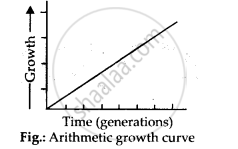CBSE (Science) Class 11CBSE
Share

# Describe Briefly Arithmetic Growth - CBSE (Science) Class 11 - Biology

#### Question

Describe briefly Arithmetic growth

#### Solution

Arithmetic growth: If the length of a plant organ is plotted against time it shows a linear curve, the growth is called arithmetic growth. In this growth, the rate of growth is constant and increase in growth occurs in arithmetic progression e.g., length of a plant is measured as 2,4, 6, 8,10,12 cms at a definite interval of 24 hrs. It is found in root or shoot elongating at constant rate. Arithmetic growth is expressed as Lt = L0 + rHere, L= length after time t. L0 = length at the beginning, r = growth rateIs there an error in this question or solution?

#### APPEARS IN

NCERT Solution for Biology Textbook for Class 11 (2018 to Current)
Chapter 15: Plant Growth and Development
Q: 3.1 | Page no. 253
Solution Describe Briefly Arithmetic Growth Concept: Growth - Growth Rates.
S